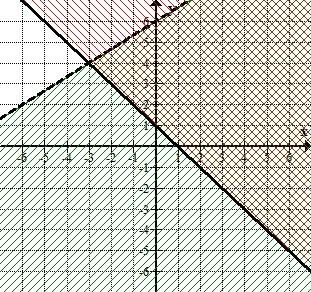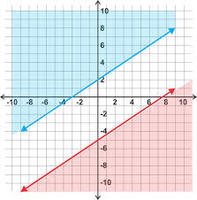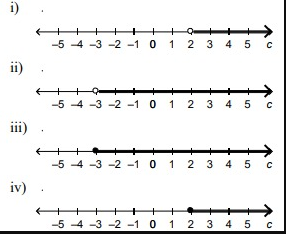# Systems of Linear Inequalities and Equations

This set of MCQs helps you brush up on important math topics and prepare you to dive into skill practice.

Start Quiz

If a system of equations has no solution, what does the graph look like?

Intersecting Lines

Parallel Lines

Skew Lines

Perpendicular Lines

What is the value of the x-coordinate to the solution to this system of linear equations?

2x + 3y = 15 and -x + 3y = 3

-5

3

-4

4

Solve for x and y.
3x+y=-5
6x+2y=10

Infinite no of solutions

No solutions

(2,4)

(4,2)

Which point is a solution?(-1,0)

(1,0)

(1,6)

(1,2)

This system has an infinite number of solutions, TRUE or FALSE?True

False

A number times 5, minus 6, is 8. Write an equation to determine the number.

5x-6=8

5x+6=8

6x-5=8

8x-6=5iii

ii

i

iv

A games room charges a \$13 entrance fee, plus \$2.35 per hour of playtime. Anne-Marie has \$29.45. For how long can she play in the games room? Choose a variable and write an inequality for this problem.

13+2.35h<_24.45

13+2.5h<_29.45

13+2.35h<_29.45

14+2.35h<_29.45

Quiz/Test Summary
Title: Systems of Linear Inequalities and Equations
Questions: 8
Contributed by: